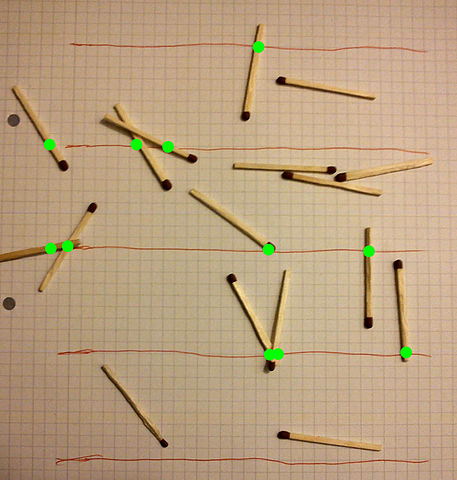# The Buffon's Needle

Calculus Level 3While messing with probability, I came across this problem:

Suppose we have a floor made of parallel strips of wood, each the same width, and we drop a needle onto the floor. What is the probability that the needle will lie across a line between two strips?

Details and Assumptions:

• Assume that you drop a needle on your rough copy and you have to find the probability that it crosses one horizontal line .

• Length of needle is equal to separation between two lines.

• Plane of needle is parallel to plane of floor or you rough copy.

×